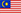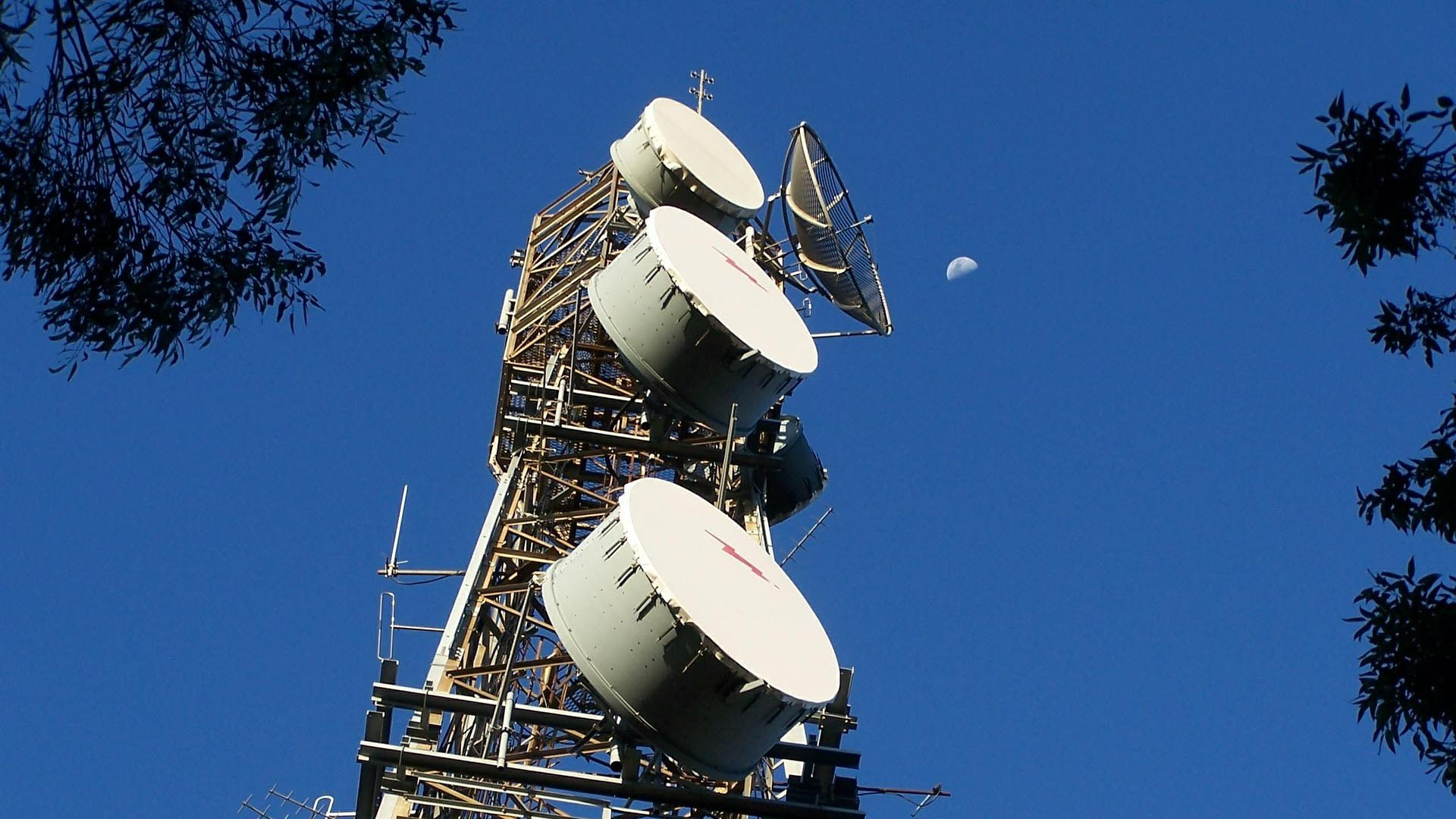MelayuBerita
How to calculation of antenna gain March 25,2020.

How to calculation of antenna gain

Expected to finish reading in 10 minutes

Gain refers to the ratio of the power density of the signal generated by the actual antenna and the ideal radiating unit at the same point in space under the condition of equal input power. It quantitatively describes the degree to which an antenna concentrates input power to radiate. The gain is obviously closely related to the antenna pattern. The narrower the main lobe of the pattern, the smaller the side lobe, and the higher the gain.

The physical meaning of gain can be understood in this way ------ To generate a signal of a certain size at a certain point over a certain distance, if an ideal non-directional point source is used as the transmitting antenna, 100W input power is required, and When a directional antenna with a gain of G = 13 dB = 20 is used as the transmitting antenna, the input power only needs 100/20 = 5W. In other words, the gain of an antenna, in terms of its radiation effect in the direction of maximum radiation, is a multiple of the input power compared with an ideal non-directional point source.

The gain of the half-wave symmetric oscillator is G = 2.15dBi. The four half-wave symmetrical oscillators are arranged up and down along the vertical line to form a vertical quaternary array with a gain of approximately G = 8.15dBi (the unit of dBi indicates that the comparison object is an ideal point source with uniform radiation in all directions).

If a half-wave symmetrical oscillator is used as the comparison object, the unit of the gain is dBd.

The gain of the half-wave symmetric oscillator is G = 0dBd (because it is compared with itself, the ratio is 1 and the logarithm is zero.) The vertical quaternary array has a gain of about G = 8.15-2.15 = 6dBd.

Several calculation formulas of antenna gain

1) The narrower the antenna main lobe width, the higher the gain. For a general antenna, the gain can be estimated using the following formula:

G (dBi) = 10Lg {32000 / (2θ3dB, E × 2θ3dB, H)}

In the formula, 2θ3dB, E and 2θ3dB, H are the lobe widths of the antenna on the two main planes;

32000 is statistical experience data.

2) For a parabolic antenna, the gain can be approximated by the following formula:

G (dBi) = 10Lg {4.5 × (D / λ0) 2}

Where D is the diameter of the paraboloid;

λ0 is the central working wavelength;

4.5 is statistical experience data.

3) For upright omnidirectional antennas, there are approximate calculation formulas

G (dBi) = 10Lg {2L/ λ0}

Where L is the length of the antenna;

λ0 is the central working wavelength;

About db, dBi, dBd and other units of the antenna

Some friends tend to confuse these units more easily. The dB values are based on logarithmic values.

(1) dB, which is simply a relative value, that is, the logarithm of the value of A over B. It is often used to say how much dB A is higher or lower than B, but it is unreasonable to say how much A's gain is dB, because we don't know what B is. It is just that many of our fellows sometimes verbally say how much dB is for the sake of simplicity, but this is not accurate enough, but often it is wrong and wrong. The default is dBi, or you can ask again.

(2) dBd, which has a standard reference value, that is, B is specified as a free-space half-wave dipole antenna. In this way, the value of A and B is a unified reference object. You tell the same good that this antenna 10dBd, He understands that your antenna can gather 10 times the energy in the main radiation direction than a half-wave dipole antenna, which is 10 times better.

(3) dBi, the meaning of this unit is relatively complicated, but it is the ratio unit that can best represent the actual environmental conditions. Here, the reference object B is a point source oscillator (there is no such object, it can be regarded as a relatively short wavelength) A small segment of oscillator, or micro-segmentation bar), in the standard definition, this point source oscillator should be an ideal spherical omnidirectional radiation, and then there is a certain mathematical relationship with dBd, that is, 1dBd = 2.14dBi. However, in fact, most antennas are affected by the installation height, the most important of which is the ground effect. Due to the mirror effect of the ground, the beam shape is often changed, and it is often 2-5dB higher in some directions. By now you should understand 19dBi. Many regular antenna manufacturers often like to use dBi to mark the gain value of the antenna. They usually indicate the installation height or the calculation method of the marked value, or what he produces is the installation environment that everyone generally knows, such as a roof-mounted antenna. Description is often omitted.

Transmit power and gain

The radio frequency signal output by the radio transmitter is transmitted to the antenna through the feeder (cable), which is radiated by the antenna in the form of electromagnetic waves. After the electromagnetic wave arrives at the receiving place, it is received by the antenna (receiving only a very small part of the power) and sent to the radio receiver through the feeder. Therefore, in the engineering of wireless networks, it is very important to calculate the transmitting power of the transmitting device and the radiating ability of the antenna.

Tx is short for Transmits. The transmitted power of radio waves refers to the energy in a given frequency range. There are usually two measures or measurements:

Power (W)-linear level relative to 1 Watt.

Gain (dBm)-proportional level relative to 1 milliwatt.

Two expressions can be converted to each other:

dBm = 10 x log [power mW]

mW = 10 [gain dBm / 10 dBm]

In wireless systems, antennas are used to convert current waves into electromagnetic waves. During the conversion process, the transmitted and received signals can also be "amplified". This measure of energy amplification becomes "Gain". The unit of measurement for antenna gain is "dBi".

Since the electromagnetic wave energy in a wireless system is generated by the transmitting energy of the transmitting device and the amplification and superposition of the antenna, it is best to measure the transmitting energy with the same measure-gain (dB). For example, the power of the transmitting device is 100mW, or 20dBm; The gain is 10dBi, then:

Total transmit energy = transmit power (dBm) + antenna gain (dBi)

= 20dBm + 10dBi

= 30dBm

Or: = 1000mW

= 1W

Every dB is very important in a "low power" system, especially remembering the "3dB rule".

Every 3dB increase or decrease means doubling or halving the power:

-3 dB = 1/2 power

-6 dB = 1/4 power

+3 dB = 2x power

+6 dB = 4x power

For example, a 100mW wireless transmission power is 20dBm, a 50mW wireless transmission power is 17dBm, and a 200mW transmission power is 23dBm.

What is an antenna?

An antenna is a conversion device or transducer between a guided wave and a free space wave.

Its basic function is to transmit and receive radio waves: when transmitting, it converts high-frequency currents into electromagnetic waves; when it receives, it converts electric waves into high-frequency currents.

Antenna gain

Under the condition that the input power is equal, the ratio of the power density of the signal generated by the actual antenna and the ideal radiating unit at the same point in space. It quantitatively describes the degree to which an antenna concentrates input power to radiate. The gain is obviously closely related to the antenna pattern. The narrower the main lobe of the pattern, the smaller the side lobe, and the higher the gain.

One of the main indicators of the antenna is the product of the directional coefficient and the efficiency, which is the performance of the antenna's radiation or reception of the radio wave. The parameters that characterize the antenna gain are dBd and dBi. DBi is the gain relative to the point source antenna, the radiation in all directions is uniform; dBd relative to the gain of the symmetrical array antenna dBi = dBd + 2.15. Under the same conditions, the higher the gain, the longer the distance the radio wave travels. Generally, the antenna gain of a GSM directional base station is 18dBi and the omnidirectional is 11dBi.

2020-3-25

rekod pesanan
•#### 2020-7-7 100pcs log periodic antenna WH-GSM-O3 ready to ship

•#### 2020-6-29 2000pcs GSM magnet antenna WH-GSM-O3 ready to ship

•#### 2020-6-23 100pcs 4G mimo panel antenna WH-LTE-D10X2 ready to ship

•#### 2020-6-15 whwireless 5G NR mimo antenna WH-5G-MM8x2 on producing

•#### 2020-6-5 3000pcs GSM 3G magnet antenna WH-GSM-O3 finished

Dapatkan Sentuhan
• Wellhope wireless Communication equipment Ltd (China):

No.8, Bidi Road Xinan Street SanShui District FoShan City, Guangdong , China

Tel : 0086 757 87722921

Whatsapp : 0086-13710314921

• Hubungi Kami

E-mel : wh@wellhope-wireless.com

E-mel : kinlu@whwireless.com

Skype : kin2008v

Hantar satu mesej

Perkhidmatan Dalam Talian

• 0086 757 87722921

• 0086-13710314921